##### Question

In: Electrical Engineering

# Draw a state diagram of the string pattern recognizer, implement it according to the design sequence...

Draw a state diagram of the string pattern recognizer, implement it according to the design sequence of the FSM, and draw a schematic diagram.

## Solutions

##### Expert Solution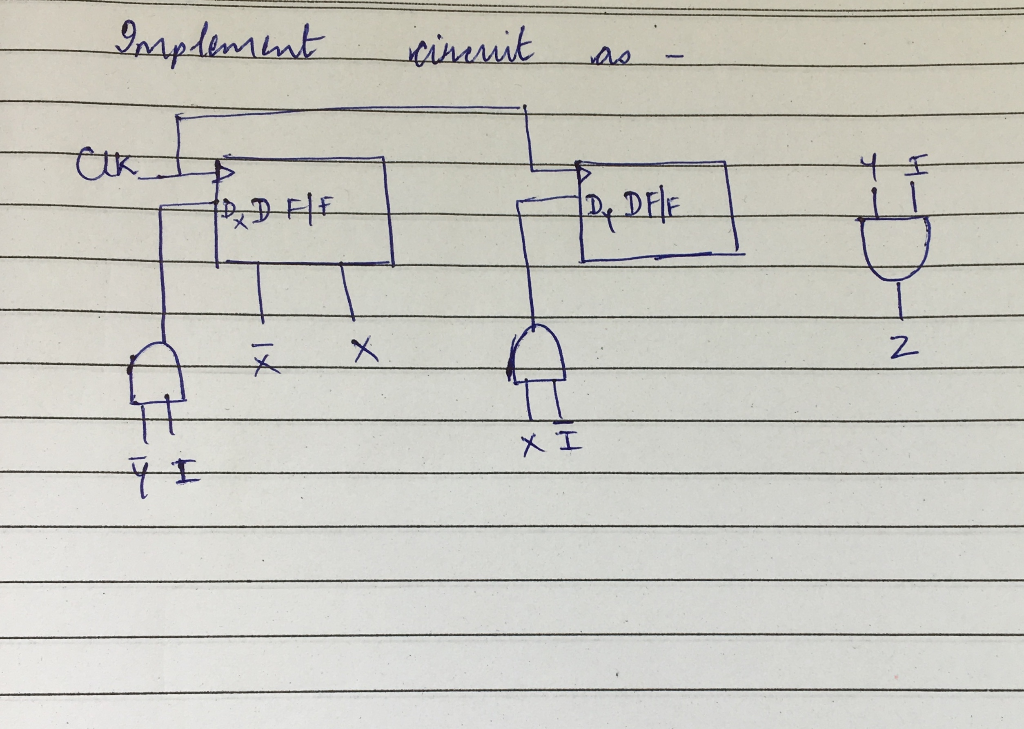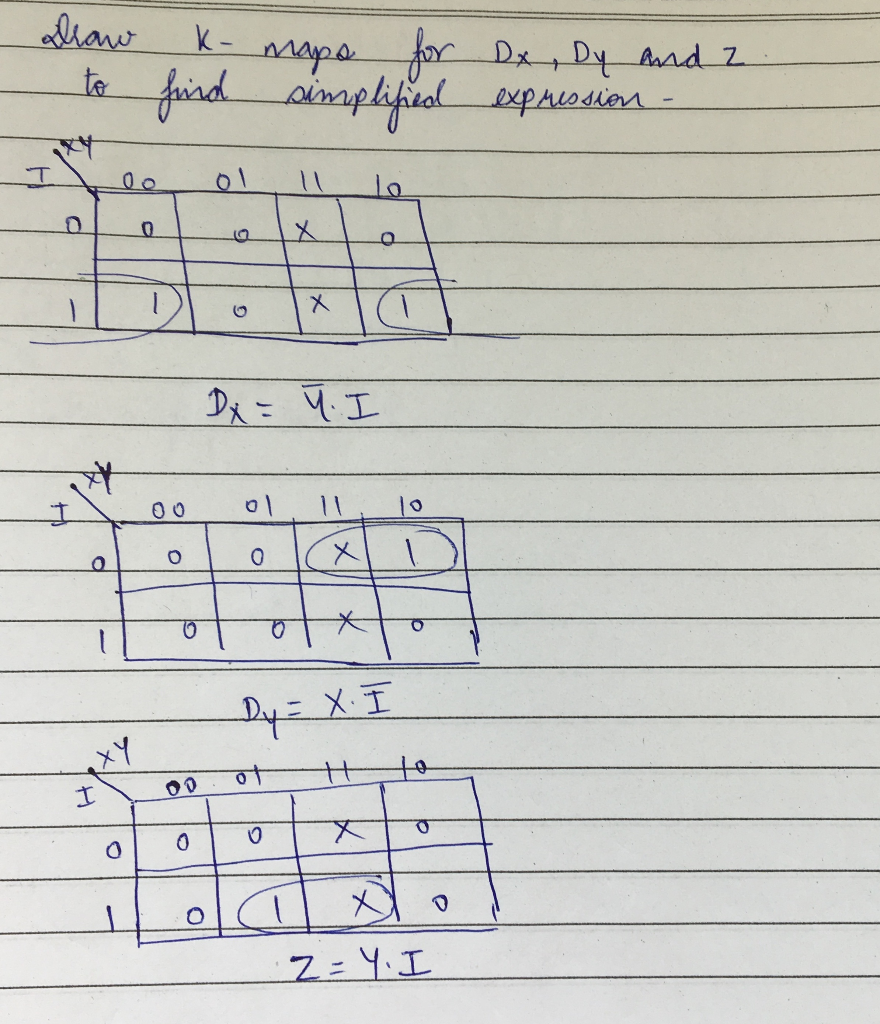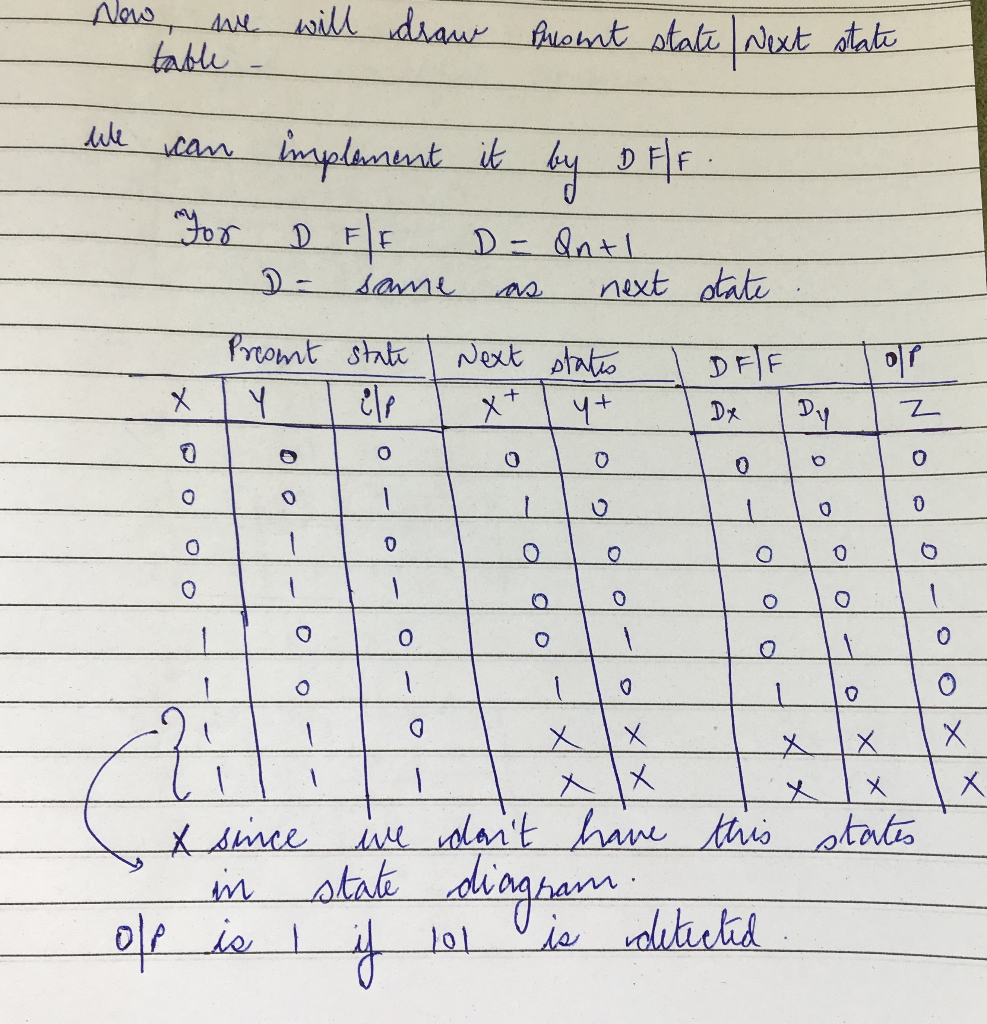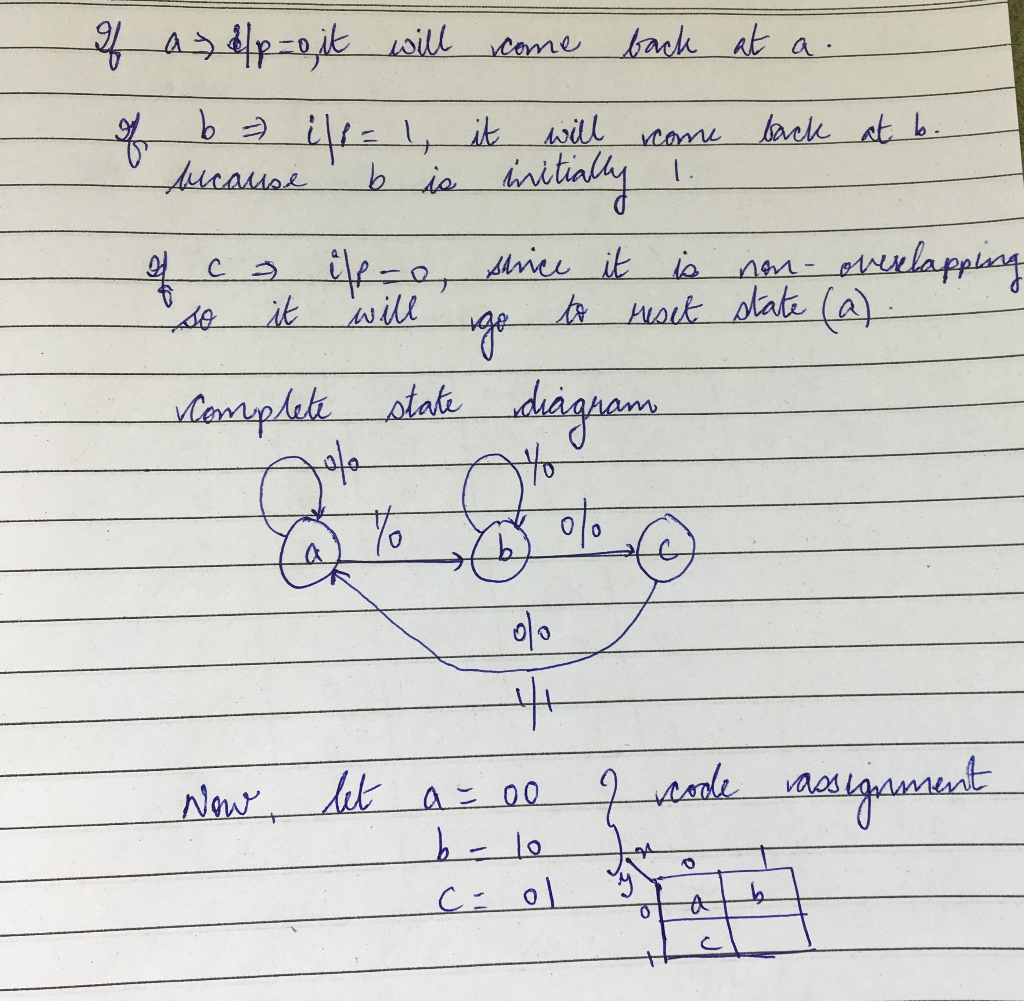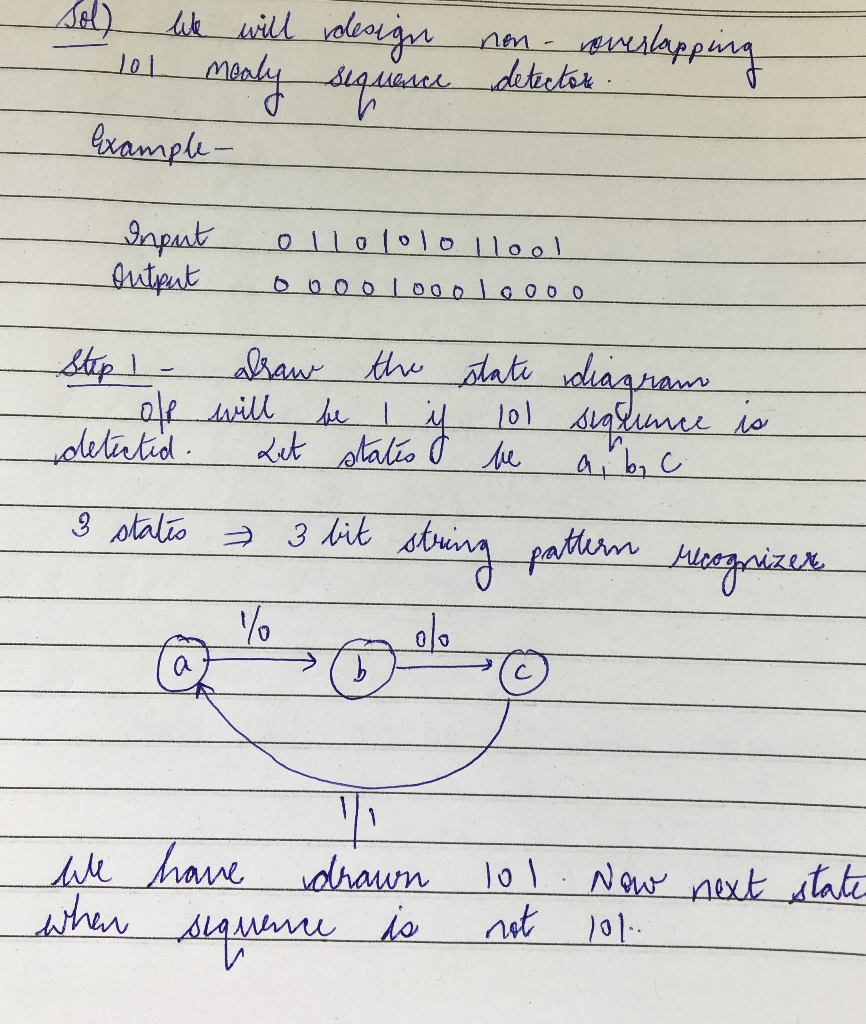## Related Solutions

##### Design a Mealy state diagram for a sequence detector that has a single input and a...
Design a Mealy state diagram for a sequence detector that has a single input and a single output. The output is to be “1” unless the input has been “0” for four consecutive clock pulses or “1” for three consecutive pulses. Implement your design using D flip-flops and any logic gates. Assume non-overlapping input sequences are to be detected.
##### Design a bus arbiter; A.) Plot the algorithm state chart. B.)Draw the state table C.) implement...
Design a bus arbiter; A.) Plot the algorithm state chart. B.)Draw the state table C.) implement suitable hardware
##### Instruction: Use Microsoft Visio to draw a use case diagram and a sequence diagram for the...
Instruction: Use Microsoft Visio to draw a use case diagram and a sequence diagram for the following business requirements. Online Recruiting System (website) 1) The Online Interview System: The search committee usually selects 3 to 5 candidates for a Skype interview. When the search committee starts a Skype interview, they connect their web browsers to an interview module developed by the company’s IT team (we call this type of software as home-developed software). The interview module then connects to Skype....
##### First derive the truth table for the requested modulus sequence, draw the circuit, then implement it...
First derive the truth table for the requested modulus sequence, draw the circuit, then implement it using only the built-in AND, OR, and NOT gates. Exercise 18 [3.0] LOCK Consider a boolean function with inputs: d, e, f, g. The value of the inputs can be defined as V = 8*d + 4*e + 2*f + 1*g. The output of this function is true if V mod 3 is 1.
##### Design an ER Diagram for the given problem. Link the tables that are related and implement...
Design an ER Diagram for the given problem. Link the tables that are related and implement your design using MySQL. Insert at least a minimum of 5 records. Ensure that the data to be added are related to other tables. Post here the screenshot of the following: Entity Relationship Diagram with attributes, PK, and FK. Use the "describe" command to view the structure of the tables. Use the "select" command to view the contents of the tables. Company ABC has...
##### Design a 5-bit binary counter using JK flip flops. Draw the flip-flop circuit diagram, the state...
Design a 5-bit binary counter using JK flip flops. Draw the flip-flop circuit diagram, the state graph, the timing diagram, the truth table (with clk pulse) and the state table (with present and next states).
##### Design a state diagram that detects the 7-bit ASCII code of the last alphabet of your full name from a sequence of incoming bits
Design a state diagram that detects the 7-bit ASCII code of the last alphabet of your full name from a sequence of incoming bits. Derive the truth table and draw the circuit diagram of this system using D-Flip Flops. Note: “Muhammad Ali” than you have to detect ASCII code for “i”. Note : Do this for "i"
##### Write a VHDL code to implement a Finite State Machine that with an 8 bit sequence...
Write a VHDL code to implement a Finite State Machine that with an 8 bit sequence input (can be any sequence, but lets say it is 11001000), determine how many states there are as well; so if the input sequence is correct it will show the number 1 in a 7 segment display, otherwise it will be 0 in the same 7 segment display. If the input sequence is incorrect, start from the beginning.
##### 1. Design a sequence detector, a Mealy finite state machine to detect the serial bit sequence...
1. Design a sequence detector, a Mealy finite state machine to detect the serial bit sequence 1101, where the most significant bit (MSB) comes first and the least significant bit comes last. A) Draw the state diagram B) Draw the state table C) The circuit is to be implemented using JK flip-flops and combinational logic circuit. Derive the Boolean expression necessary for this implementation. D) Sketch the circuit diagram for your design. This should show all the flipflops, logic gates...
##### Java String search Design and implement a recursive version of a binary search.  Instead of using a...
Java String search Design and implement a recursive version of a binary search.  Instead of using a loop to repeatedly check for the target value, use calls to a recursive method to check one value at a time.  If the value is not the target, refine the search space and call the method again.  The name to search for is entered by the user, as is the indexes that define the range of viable candidates can be entered by the user (that are...